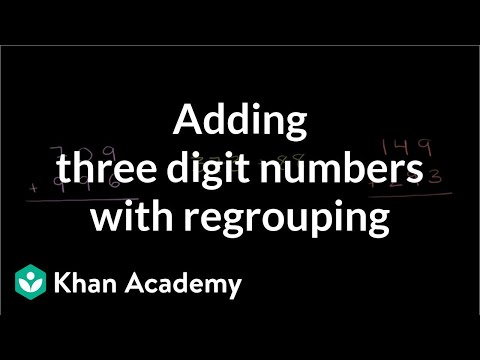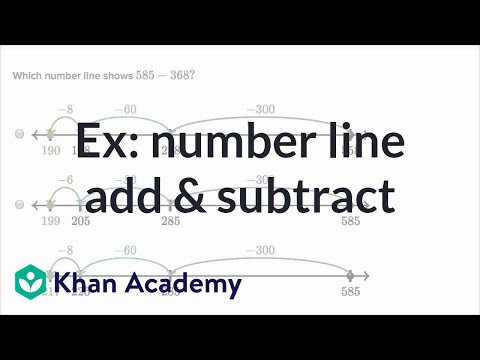# Worked example: Subtracting 3-digit numbers (regrouping twice) (Full video)

Description: Sal using regrouping (borrowing) to subtract 913 - 286. Created by Sal Khan. This 1 in the tens place represents 10. So let's try to subtract going place by place. So if we start in the ones place, we have a problem immediately.

### Other videos you might be interested in### Adding 3-digit numbers with regrouping (Full video)